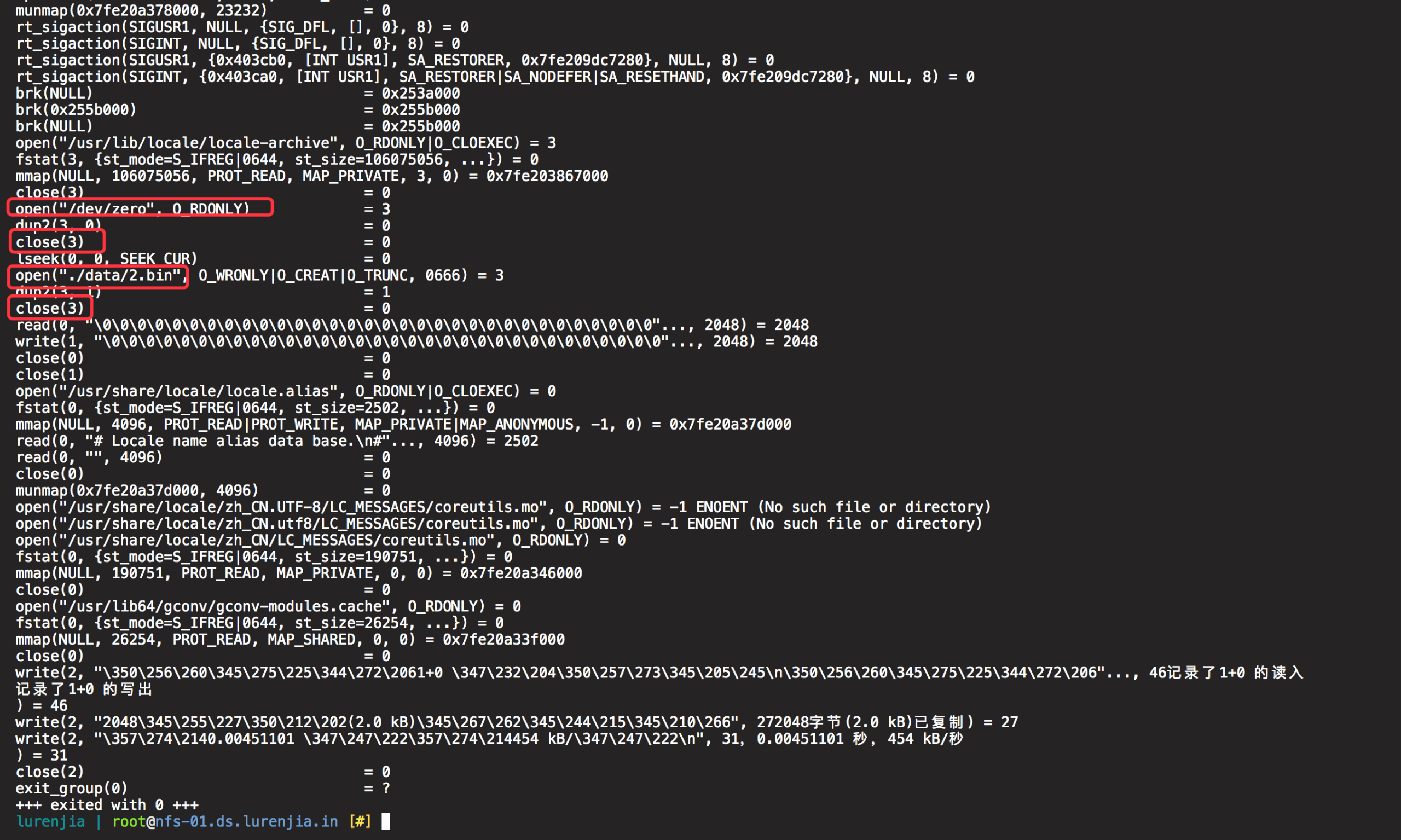## 0x01 笨办法

``````mkdir data
for ((i = 1; i <= \$1; i++)); do
dd if=/dev/zero of=./data/\${i}.bin bs=\${2}k count=1 &>/dev/null
done
``````

1. 生成的文件个数
2. 生成的文件大小(KB)

``````./gen_v1.sh 10000 16
``````

## 0x02 另一个笨办法

``````mkdir data
for ((i = 1; i <= \$1; i++)); do
dd if=/dev/zero of=./data/\${i}.bin bs=`shuf -n 1 -i \${2}-\${3}`k count=1 &>/dev/null
done
``````

1. 生成的文件个数
2. 生成文件的最小体积(KB)
3. 生成文件的最大体积(KB)

``````./gen_v2.sh 10000 4 32
``````

## 0x03 巧办法``````mkdir data
count=\$1
size=\$2
total_size=`expr \$count \* \$size`
dd bs=\${total_size}k count=1 if=/dev/zero | split -b \${size}k -a \${#count} -d - "./data/"
``````

1. 生成的文件个数
2. 生成的文件大小(KB)

``````./gen_v3.sh 1000 1024
``````

## 0x04 不那么巧的巧办法

``````mkdir data

count=\$1
min_size=\$2
max_size=\$3
file_block_size=4096
ratio=`echo "\$file_block_size / ((\$min_size + \$max_size) / 2)" | bc`
iter_count=`expr \$count / \$ratio`

for ((i = 1; i <= \$iter_count; i++)); do
dd bs=\${file_block_size}k count=1 if=/dev/zero | split -b `shuf -n 1 -i \${min_size}-\${max_size}`k -a 3 -d - "./data/\$i-"
done
``````

1. 生成的文件个数(近似值)
2. 生成文件的最小体积(KB)
3. 生成文件的最大体积(KB)

``````./gen_v4.sh 10000 4 32
``````

``````file_true_count=`echo "\`ls -l data | wc -l\`-1" | bc`
count_diff=`echo "\$file_true_count-\$count+1" | bc`
cd data && rm -rf `ls -l ./ | tail -n \${count_diff} | awk '{print \$9}' | tr "\n" " "
``````